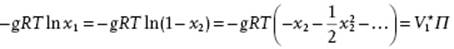﻿ ﻿Colligative Properties - Mixing Liquids: Molecular Solutions - Liquid-State Physical Chemistry: Fundamentals, Modeling, and Applications (2013)

## Liquid-State Physical Chemistry: Fundamentals, Modeling, and Applications (2013)

### 11.3. Colligative Properties

So far, we have seen that the vapor pressure of solutions deviates from that for pure liquids. Solutions also show other deviations from pure liquids, for example, freezing point depression. To analyze this effect we assume that the solid solvent is a pure phase. Then, since at the freezing point the chemical potential of the solvent (component 1) is equal in the solid (s) and liquid (l) phases, we have

(11.30)where ΔfusGμ1(l) − μ1(s) is the Gibbs energy of fusion. Differentiation with respect to temperature and using the Gibbs–Helmholtz equation ∂(μ/T)/∂(1/T) = Hm yields

(11.31)Upon integration, this equation from pure solvent conditions (a1 = 1, T = T*) to arbitrary values of a1 and T, we obtain

(11.32)which can be used to calculate the activity of the solvent in a solution. An approximation to Eq. (11.32) for sufficiently dilute solutions is obtained by using

(11.33)Assuming that ΔfusH is independent of T over the range T* to T, this results in

(11.34)or, since T−1T*−1 = (T* − T)/TT* ≅ ΔT/T*2, in the freezing point depression

(11.35)A similar reasoning for boiling point elevation leads to a boiling point T* + ΔT, where T* is the boiling point of the pure substance and ΔT = Kboix2R(T*)2x2vapH. Although the temperature effects are small, the freezing point depression and boiling point elevation can be used to determine the molecular mass of the solute. Table 11.2 provides data of Kfre and Kboi for some solvents.

Table 11.2 Cryoscopic constants Kfre and ebullioscopic constants Kboi for some compounds.a)

 Compound Kfre(K kg mol−1) Kboi(K kg mol−1) Acetic acid 3.90 3.07 Benzene 5.12 2.53 CS2 3.8 2.37 CCl4 30 4.95 Naphthalene 6.94 5.8 Phenol 7.27 3.04 Water 1.86 0.51 Camphor 40 –

a) Data from Ref. .

Since in the dilute approximation the effects are only dependent on the number of solute molecules (and the type of solvent), these effects are denoted as colligative properties. Another colligative property is the osmotic pressure. This effect can be observed if we have a semipermeable membrane, that is, a membrane which is permeable to only one component (usually the solvent), with on one side the pure solvent at pressure P0 and on the other side the solution at pressure P. This configuration leads to an excess pressure, normally addressed as osmotic pressure Π = PP0. The reasoning is similar as for the freezing point depression, and starts by equating the chemical potential of the pure solventto that of the solution μ1, so that we have

(11.36)Since ∂μ1/∂P = V1, we may write

(11.37)(11.38)If we compare this expression with, we have

(11.39)where the second step can be taken if we assume that the partial volume V1 can be approximated by the molar volume of the pure solventand that the solution is incompressible. This yields an explicit expression for the activity of the solvent. Applying the volume and incompressibility approximation to Eq. (11.38), we obtain

(11.40)which reduces, usingwith g the osmotic coefficient, to

(11.41)This expression explains why g is denoted as osmotic coefficient. For an ideal solution g = 1, and for x2 → 0 the expression reduces to the van't Hoff law

(11.42)Alternatively, the use of ϕ leads to. For real solutions g ≠ 1, and the easiest way to incorporate the effect is to write g as a power series in x2 reading(11.43)The coefficients C2, C3, … depend on the nature of the solute and solvent and, of course, on T and P. Note that the g-expansion (or osmotic virial expansion) starts with the term 1, because the limiting van't Hoff law should be recovered for c2 → 0. The osmotic effect is large, for example, for seawater with ∼35 g l−1 NaCl or c2(NaCl) ≅ 0.6 m, Π ≅ 27 bar, to be compared with a freezing point depression of ∼2 K for the same molarity. By using the osmotic pressure effect, the molar mass M2 of the solute molecules can be determined by using a concentration series with decreasing c2. The intercept of a plot of Π/w2 versus w2, where w2 = c2/M2 is the weight fraction, provides M2, independent of non-ideality. Normally, there is a distribution of molar masses, and since each component contributes equally to the sum, the mass so determined is the number average. For polymers, a molar mass up to 2 × 10 kg mol−1 can be determined since quite low molarities still yield measurable pressure differences. For increasing molar mass, however, the decreasing intercept RT/M2 limits the accuracy.

Example 11.2

In the table below, the osmotic heights h at 298 K of various molarities c of poly(vinyl chloride) (PVC) in cyclohexanone (ρ = 0.98 g cm−3) are given. The pressures are thus Π = ρgh, where g is the acceleration due to gravity.From a graph of h/w2 versus w2, the intercept I = RT/ρgM2 is calculated as 0.21. Therefore, M2 = RT/ρgI = 128 kg mol−1.

﻿